Home

# General Relativity – part 2

We now come to the most interesting and probably the least understood aspect of General Relativity (GR).  And that is GR’s explanation of how gravity works and how it determines the motion of orbiting bodies.

GR is often described in terms of space bending or space curvature.  The idea being that a massive body curves the space around it and this affects the motion of other bodies nearby, including light.  In reading the literature, one often finds expressions such as “orbiting bodies travel in straight lines though curved space” or “light moves along the shortest path between two points, which will be a curve in the presence of mass.”

A popular explanation for how this works is the rubber sheet analogy.  This involves a bowling ball making a depression on a trampoline and a marble either rolling around inside the depression or rolling directly into it.  Here the bowling ball represents a planet, the marble a satellite, and the rubber sheet is space being bent through an additional dimension.

These explanations can be confusing.  If space was curved and orbiting planets were following a straight path through curved space then we would expect the curvature to be the same for all objects regardless of their speed.  In other words, an object should follow the same orbit path irrespective of whether it was going fast or slow.  But we know that doesn’t happen.

In the case of the stretched rubber sheet, a frequent objection is that that it requires gravity to make it work in the first place.  Without gravity there would be no ‘down’ and the marble would have no reason to move at all.  In other words the analogy is using gravity to explain gravity.  Another problem is that the marble would create its own depression which means it would be unaware of the bowling ball’s depression and thus wouldn’t roll into it.

Such confusion is understandable and comes from the way GR theory is being expressed.

Well here’s some surprising news: General Relativity has nothing to do with space curvature.  Not space bending.  Not time bending.  Not even ‘space-time’ bending.  Under GR, the distance between two points remains the same regardless of whether gravity is present or not.  What GR does have everything to do with is time dilation

Here’s how it works.  Under GR an orbiting body moves according to the principle:

A body moves along the path that makes time dilation a minimum.

That’s it!  So what does this mean?  Let’s take a simple example.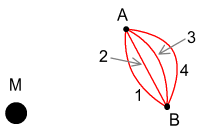In the diagram above we have a large mass M and a small body moving past it.  The large mass could be the Sun or a planet.  And the small body could be a moon or grain of sand for example.  We’ll say the small body wants to get from A to B and needs to do so in a fixed amount of time.  There are any number of paths it could take and any number of variable speed changes it could make along the way.

According to GR it needs to get there with the least amount of time dilation.  Now recall from the previous chapter and the chapter on the Lorentz transform that time dilation increases the faster we go and increases the closer to a mass we get (i.e. it increases in higher gravity). We want to reduce both of these.  In summary:

Slow = good.  Fast = bad.  Low gravity = good.  High gravity = bad.

In other words we need to get from A to B as slowly as possible but also while being as far away from M as possible.

In the above diagram four sample paths are shown.  The simplest is path 2 – the straight line.  This will satisfy the condition of making the journey as slow as possible.  Unfortunately path 2 also means that most of that time is spent nearby M, in high gravity, and that’s undesirable.  So we’ll try path 4.  This one takes the object far away from M, reducing gravitational time dilation.  Trouble is, path 4 also requires moving at high speed because the path is long and this increases movement-based time dilation.  As for path 1, that increases dilation of both types.  So it is definitely ruled out.  The correct path turns out to be 3, giving the right balance between the two types of dilation and giving the minimum possible amount of time dilation over all.

The best path will depend on the total time allotted in moving from A to B.  With less time available, a straighter path is best because it reduces average velocity.  If a large amount of time is allotted, we will instead choose a path more like 4.

All of this seems to be in agreement with the classical prediction of gravity for orbital motion.  And it turns out that the ideal path chosen will in fact be the same as the one predicted by Newton’s laws, with some minor differences to be discussed later.

#### The Schwarzschild Metric

General Relativity usually involves lots of complicated tensor calculus and confusing symbols.  Because of this it is best to avoid delving into its specifics until a proper understanding can be gained at a conceptual level.  Basically however, GR is built around something called Einstein’s field equations and these fully describe the effects of gravity and orbital motion.  The solution to the equations involves solving multiple differential equations simultaneously and is very difficult except in simple situations.  The earliest and best known of these is Karl Schwarzschild’s solution for a point mass.

The ‘Schwarzschild Universe’ (to give it a name) consists of a single point mass and no mass elsewhere.  Although there may be massless particles nearby.  For the sake of understanding we can say this universe contains a special clock that measures ‘universal time’.  This clock is motionless, with respect to the point-mass, and located in a special ‘gravity-free’ zone.  This clock therefore runs faster than all other clocks elsewhere because other clocks will be experiencing gravity which will slow them down.  And they might also be moving which will slow them further.

We will also say there is massless spacecraft that moves about near the point-mass.  This spacecraft carries its own on-board clock which will run slower than the universal clock.

Let the point-mass have a mass of M, the universal clock run in increments of dt, and the spacecraft’s clock run in increments of ds.  There is a formula that describes exactly how ds relates to dt called the Schwarzschild Metric .  It is: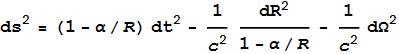---- (1)

Where (alpha) α = 2GM/c2, R is a function of distance to the point-mass (see below), dR is an increment of R (a movement away from the point-mass), and is an increment in distance tangential to the radial direction (a sideways movement) .

R is described by this formula R = (r3+α3)1/3.  Where r is the distance to M.  For small values of α (which is normally the case) Rr.  For very large values of M and small r however, we can’t use that approximation.  This becomes important for the black-hole situation because it turns out that 1-α/R can never become zero without r becoming zero.  Hence black-hole singularities cannot occur under the proper description of GR .

Let’s now look at what equation (1) is saying.  We’ll start by dividing both sides by dt2, and noting that dr/dt represents radial velocity and /dt represents tangential velocity.  We will also replace R with r as a good approximation.  Taking the square root of both sides then gives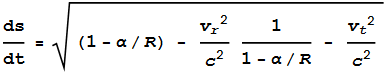---- (2)

Where vr is radial velocity (velocity directly away from M) and vt is tangential velocity (velocity running sideways to M).  Using the relation that

1/(1-b) = 1 + b + b2 + b3 + … ≈ 1+b   (for small b)

and setting b=α/R, we can closely approximate (2) to---- (3)

In most cases vr<<c and we can ignore the vr2/c2 term.  Although this term will have importance in special cases as will be discussed later.  For now though, we’ll assume vr/c=0.  We can also substitute the simple Pythagorean sum of tangential velocity addition, that is vr2+vt2=v2 where v is the net velocity.  This makes equation (3)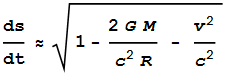---- (4)

The above should now start looking familiar.  Notice that when we set M=0 (a zero gravity environment) we get---- (5)

This becomes the Lorentz Transform!  It tells us how much slower ds runs behind dt when the spaceship containing clock ds is moving with velocity v.  Actually the Lorentz Transform is expressed as the reciprocal of this because it describes dt/ds.

Next we again take equation (4) but and this time set v to zero.  We get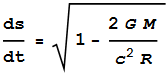---- (6)

And this is the equation for gravitational time dilation as shown in the previous chapter.

So what the Schwarzschild Metric tells us is the amount of time dilation that will be experienced by a massless body in the proximity of a point-mass based on a combination of the point-mass’s gravity and the particle’s motion.

#### What is velocity relative to?

We’ll pause here for a closer look at the situation.  In earlier chapters we looked at Special Relativity (SR) and noted one of its main problems is we don’t know what velocity should be measured against.  Velocity is considered a relative quantity under both SR and classical mechanics.  Therefore in order to calculate velocity-based time dilation it is necessary to know what velocity is relative to.  In the case of SR it is ambiguous.

But this point-mass scenario would seem to solve that.  Here, all velocities are measured relative to that mass.  Now look again at equation (5).  There we eliminated the mass by setting M=0 and got the Lorentz transform.  The mass is gone but we are still measuring velocities relative to it!  How can that be?  Surely the velocity term no longer has any meaning.

It gets more interesting.  Instead of making M equal to zero we’ll slowly decrease its value until it becomes insignificantly small, like a microgram.  And we’ll say the distance to our massless spacecraft is the Earth-Sun distance.  Despite M being so small and having so little gravity, we still fully base our velocities on it.  This doesn’t seem right.  Alternatively we keep M the same and increase the radial distance of our orbiting body to something extreme like a billion light years.  At this distance M’s gravity is so low it could never be detected and the point-mass may as well not exist.  Yet despite this, we again use that mass to measure velocities against.

Something is amiss here.  No one should seriously suggest that a mass so small or far away could influence time dilation calculations by any measurable extent.  If GR were to be more credible it should include a function in front of the v2/c2 term that decreases with lower mass and greater distance.  This appears to be a limitation in the GR theory that either goes unnoticed or is conveniently overlooked.

#### Where's the acceleration?

Another oddity of the Schwarzschild Metric is that it doesn’t mention acceleration.

Recall that when deriving the time dilation aspects of gravity, the first step was to determine how much dilation would result from acceleration.  Once having a suitable formula for acceleration, we then substituted acceleration for gravity and arrived at an equivalent formula for gravity.  So we have our time dilation formula for gravity.  But having achieved this, for some reason we now regard the acceleration formula as unimportant.

When planets orbit the Sun they are constantly changing direction and hence constantly experiencing acceleration.  If acceleration has an effect on time dilation we should therefore expect the Schwarzschild Metric to somehow account for it by including terms for acceleration.  If the Metric did include acceleration terms they would appear as d2R and d2Ω .  But such terms are clearly absent.  Why so?

One problem of including acceleration terms is that the predictions for orbital motion will be wrong.  This however, is not a valid reason for their exclusion.  Rather, it indicates that either the Metric is wrong or that the fundamentals of GR are wrong.

We’ll now turn to a more interesting aspect of GR.  This is where GR makes predictions different from what classical mechanics would make.  The first of these is light-bending.

If we were to assume that Newtonian gravity affects light the way it affects regular matter, we could calculate the degree of bending a light-ray would experience as it passed by a massive object such as the Sun.  GR also predicts light will be bent but it predicts double the amount Newtonian gravity would.  Why is this?

Go back to equation (3).  If we take this and include the net velocity relationship, vr2+vt2=v2 (as was included in equation (4)), we get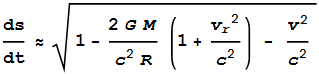---- (7)

Notice the  vr2/c2 term.  In equation (4) we removed this because it was small for orbiting planets and satellites.  But for light itself it will be large.  As a ray of light skims past the Sun, vr2 will be very close to c2.  That means the term inside the parentheses becomes almost equal to 2.  And we get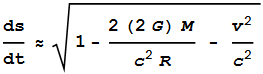---- (8)

Without going through the complicated math, what we have effectively done is double the value of the gravitational constant.  This equates to doubling the ‘force’ on the light ray and we end up with twice the bending.  There’s obviously more to it than that but that’s the basic idea.

The next prediction GR makes that is at odds with Newtonian gravity has to do with elliptical orbits gradually changing their orientation.  This is known as ‘advancement of perihelia’ and becomes noticeable for planets with stronger elliptical orbits, such as Mercury.  What makes this happen?

In an elliptical orbit a planet spends some of its time moving toward and away from the Sun.  That means the radial velocity vr is non-zero.  We’ll take equation (7) and rewrite it in terms of vt and vr.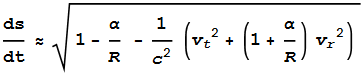---- (9)

We want to make (9) a maximum, i.e. make time dilation a minimum.  We already know this requires reducing overall velocity (velocity = bad).  What (9) tells us is that radial velocity has a slightly greater impact on dilation than tangential velocity (radial velocity = slightly badder).

Another thing (9) tells us is that the impact of radial velocity becomes greater with smaller values of r (closer to the Sun) and its impact is reduced as the planet moves farther away.  Therefore, in order to reduce time dilation, the planet will need to adjust its orbit to accommodate this.  This can be done by reducing its outward velocity when it is close to the Sun, then increasing it as it moves farther away.  The overall result is this:The grey line shows what Newtonian gravity predicts.  The black line is what GR predicts.  As the planet begins moving away it slows down, making it closer to the Sun, and then speeds up as it gets farther away.  Here the blue dot indicates a point that has moved closer.  The result is the far-point of the ellipse (the aphelion) is shifted along slightly.  A similar thing happens on the return trip in which the near point of the ellipse (the perihelion) advances slightly with each orbit.

Again there’s obviously more to it than that and the math is very complicated.  But that’s the basic idea.

#### Another Equivalence problem

The fact that GR predicts a different amount of bending for light presents another problem for the Strong Equivalence Principle.  This principle claims that if you were sealed inside an elevator you wouldn’t know if it were sitting motionless on Earth or accelerating through outer space at a rate of 9.8 m/s2.

But if the above GR prediction is true then it appears there’s a way after all.  Simply shine a laser across the elevator.  If the light bends in a manner predicted by Newton, you’re in space.  If it bends with double that amount, you’re on Earth.  This means that, according to GR theory, gravity is not equivalent to acceleration after all.

#### Vertical motion

Most of the discussion so far has been about orbital motion.  That is, bodies moving around a point mass at periodic intervals.  But GR can be applied to any type of motion such as vertically falling or rising objects.

Suppose you threw a stone directly upward.  Experience tells us that the stone will begin its journey at full speed, gradually slowing to a stop, then falling again.  Classical mechanics tells us that its motion will be a parabola when plotted against time.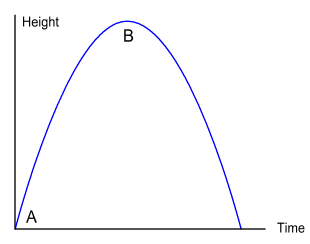It’s interesting to note that GR predicts the same thing.  If we label your hand as point A, the top of the parabola as point B, and specify the time it takes to get there, GR also predicts the stone will slow as it moves upward.  Why it that?

Let’s say instead of a stone you were shooting a high-speed projectile several hundred km into space and there was no atmosphere to slow it.  GR aims to minimise the extent of time dilation.  When the projectile is farther from Earth, gravity is lower, and hence time dilation is lower.  So GR ‘decides’ to have the projectile spend most of its time farther away.  To do this the initial velocity is set high and the ending velocity is low.

Without going through the maths it turns out that the ideal solution for least amount of dilation is also the classical mechanics prediction (ignoring the microscopic GR adjustments described earlier).  And for a falling object the reverse will be true.

The interesting question here is how is GR able to distinguish between minor differences in gravity?  For an object rising hundreds of km above Earth, this is easy because gravity drops measurably.  But what about throwing a stone upward by a few inches – how can GR detect any difference in gravity over that small distance?  If there is no difference in gravity over a small range then presumably GR would keep velocity constant.

Of course there must be some difference in gravity over any vertical distance.  And presumably GR is somehow detecting it and taking it into account.  Fortunately there is a way to determine whether that is the case.  Above the Earth’s surface, gravity decreases with altitude.  But below the surface the opposite happens.  That is, gravity decreases with depth, becoming zero at Earth’s centre.

So the test is this:  Stand inside a mine shaft and throw a stone upward.  As the stone moves upward it experiences an increased downward pull.  This is the opposite of what you get above ground.  If GR were based on subtle gravitational differences then we would expect it to require the stone to begin slowly and increase speed toward the top.  But we don’t see this.  Instead the stone moves in much the same way as it does above ground, i.e. in a parabola.  This would suggest that GR is not responsible for the stone’s motion after all. 

#### The stationary particle

We’ll now look at a situation that GR genuinely has problems with.  Suppose you observed a particle to be situated some distance away from Earth.  Your observation was made using high-speed film and the photograph gave no clue about which direction the particle was moving because there was no blur.  Several hours later you took another photo and found the particle at the same location.  Based on your understanding of gravity you would know that it is impossible for the particle to have been sitting there the whole time.  So you would conclude that the particle must have either orbited the Earth and returned to the same point, or was earlier moving upward and had then fallen down to that same point.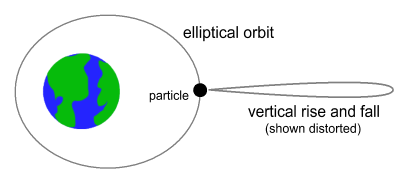GR would also draw the same conclusion and say the particle must have taken one of those two paths.  Problem is you wouldn’t know which.  In both cases, classical and GR, you’d be lacking information.

If you want to know how a particle orbits using classical mechanics all you require are its ‘initial conditions’.  This information consists of the particle’s starting position and velocity.  With this information the force on the particle can be calculated and incremental steps in its position can be mapped into a complete orbit.

With GR it’s a bit different.  To know a particle’s path we need both a start and end location and the time taken to get there.  Knowing the start point is easy because that’s what we begin with.  But the end-point and time taken... how can these be known in advance?

Under GR a particle’s initial condition consists of position only.  We don’t care about velocity.  But let say we knew both position and velocity.  Using that position and velocity, and ignoring the acceleration predictions of Newtonian gravity, we can calculate the first step in its journey for a small increment of time.  That gives us two points in the orbit.  From then onward we can use GR to calculate additional steps.  Each additional step will be determined such that it, and the two steps before it, give the minimum amount of time dilation possible.  It’s a complicated process but certainly doable.  In this way a GR-based orbit can be determined based on initial conditions only.

But let’s say the particle was initially at rest.  In this case we won’t know what the second point in the orbit is because zero initial velocity means no initial movement.  Because of this GR can’t tell us what any of the following points will be.  This isn’t a problem under classical mechanics because Newtonian gravity predicts a downward acceleration and we can determine the second point in the orbit will be underneath the first.  Presumably then we could use both velocity and Newtonian gravity to calculate this second point for GR also.  However this would be cheating because we are not supposed to use Newtonian gravity theory: GR is supposed to fully determine the motion based on time dilation aspects alone.

This is not to say that GR would permit the particle to stay still.  Certainly not.  GR would require it to move in order to minimise time dilation.  Problem is, GR cannot tell us how a stationary particle should begin moving.  This is a definite failing in GR theory.

But let’s get back to the case where a particle did have some initial motion.  In that case we used its initial velocity to determine the second point in the orbit.  Here again though, we are stepping outside of GR in order to compute the continuation of an orbit.  Strictly speaking we should not be using any part of classical mechanics because GR is supposed to fully account for the path on its own.  In fact, the Schwarzschild Metric is often used to derive classical laws such as the conservation of energy and angular momentum.  This derivation would have no validity if it required classical laws as part of the input.

#### Questioning the motion

This leads us to some important questions about what GR is describing.  Under classical mechanics a body can only respond to the forces that are acting upon it at the time.  It can’t base its motion on something that it will encounter later on.  Yet GR seems to describe a body doing exactly that.  Namely that a body determines a path to a destination that it doesn’t yet know of, based on conditions it has not yet encountered.  How is this possible?

Given that it’s not possible to reverse the roles of cause and effect, GR cannot be acting as a predictor for what is about to happen.  Rather, it acts as a verifier for what has already occurred.  What GR says is: show me an orbit path segment and I’ll tell you whether it’s valid or not.

Based on this, it is safe to say that GR is not governing the motion of orbiting bodies.  Rather, a separate process must be responsible for determining how bodies move in the presence of a gravitational field.  We know that Newtonian gravity can accurately account for the majority of such motion.  But Newtonian gravity has limitations, such as not explaining perihelion-advancement.

Thus, rather than replace Newton’s gravity equation with GR, it would seem wiser to retain and make adjustments to it.  In order that the cause and effect principle is not violated these adjustments should describe forces that are solely determined by the information that is able to be ‘felt’ by the orbiting body at the time.

#### Conclusions

The acceleration time dilation aspects of General Relativity (GR) are internally self-contradictory and thus could not be true.

The Strong Equivalence Principle between gravity and acceleration fails because the time-dilation formula for gravity includes a body’s radius in addition to its field strength.  It also fails because GR predicts a different degrees of bending for light in a gravitational field versus an equivalent accelerating reference frame.

The Weak Equivalence Principle contains no information that is not already contained in the Newtonian description of gravity.

GR can accurately describe the path of a free-moving body in the presence of a gravitational field and can do so with greater accuracy than Newton’s gravity equation.

GR seems illogical in determining how relative velocity is measured; especially when small masses or large distances are involved.

GR cannot predict an orbit-path in advance.  It can only verify whether a potential orbit-path is valid.

GR cannot determine how a motionless body should accelerate in the presence of a gravitational field.

 Schwarzschild’s original paper in English  http://arxiv.org/PS_cache/physics/pdf/9905/9905030v1.pdf
 is normally expressed in terms of spherical coordinates R, ϑ and φ.
 After dividing both sides of (1) by dt2 these will become the familiar acceleration  terms d2R/dt2 and d2Ω/dt2.
 See this site for a detailed discussion of why GR forbids black-hole singularities  http://sjcrothers.plasmaresources.com
 Due to Earth’s the high-density core and low-density crust, gravity below the surface initially increases with depth.  In actuality you’d need to go down several thousand km before the gravity dropped below its surface strength.  But if you did throw a stone upward from such a depth, there’s no reason to believe it would speed up as it moved away from your hand and into stronger gravity.